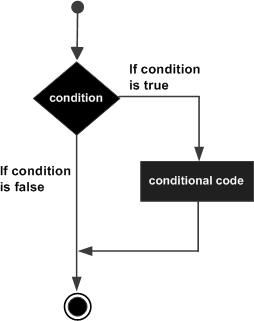# Lua - If statement

An if statement consists of a Boolean expression followed by one or more statements.

## Syntax

The syntax of an if statement in Lua programming language is −

```if(boolean_expression)
then
--[ statement(s) will execute if the boolean expression is true --]
end
```

If the Boolean expression evaluates to true, then the block of code inside the if statement will be executed. If Boolean expression evaluates to false, then the first set of code after the end of the if statement(after the closing curly brace) will be executed.

Lua programming language assumes any combination of Boolean true and non-nil values as true, and if it is either Boolean false or nil, then it is assumed as false value. It is to be noted that in Lua, zero will be considered as true.

## Flow Diagram## Example

```--[ local variable definition --]
a = 10;

--[ check the boolean condition using if statement --]

if( a < 20 )
then
--[ if condition is true then print the following --]
print("a is less than 20" );
end

print("value of a is :", a);
```

When you build and run the above code, it produces the following result.

```a is less than 20
value of a is : 10
```
lua_decision_making.htm